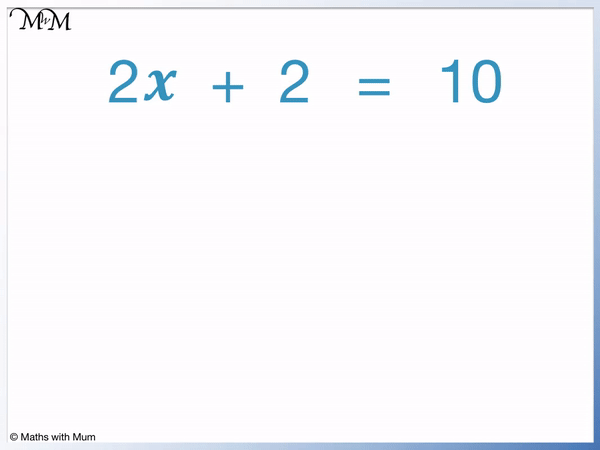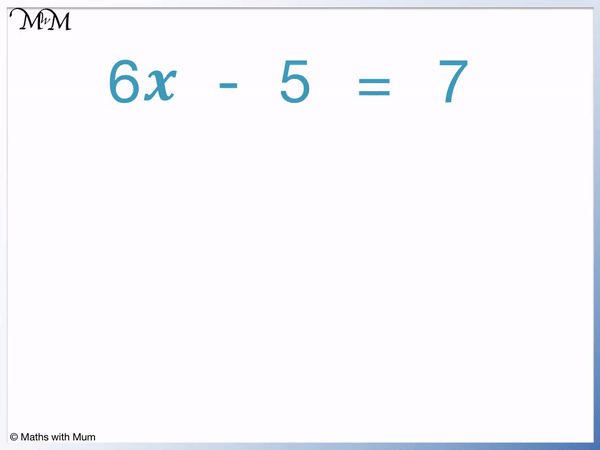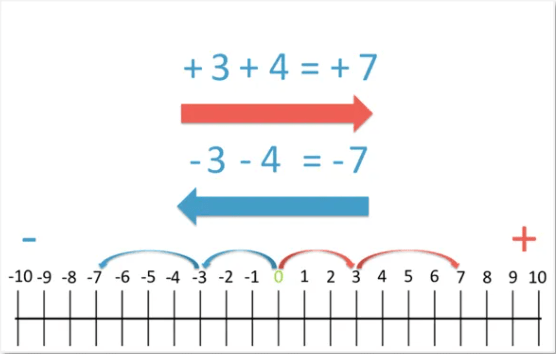# How to Solve 2-Step Equations

How to Solve 2-Step Equations• 2𝑥 + 2 = 10 is an example of a 2-step equation.
• A 2-step equation is an equation that can be solved using 2 calculations.
• The first step is to remove the number that is added or subtracted to the variable. We will remove the ‘+ 2’.
• We subtract 2 from both sides of the equation to get 2𝑥 = 8.
• The second step is to get the variable on its own without any numbers in front of it. We will remove the 2 from in front of the 𝑥.
• We divide both sides of the equation by 2 to get 𝑥 = 4.
• When the variable 𝑥 is alone on one side of the equation, we have solved it. 𝑥 = 4 is the answer.
• We can check the solution by substituting it back into the original equation of 2𝑥 + 2 = 10 in place of 𝑥. The left hand side of the equation becomes 2 × 4 + 2, which equals 10. Since both sides of the equation are the same, the solution is correct.
Add or subtract the same number to both sides of the equation. Then multiply or divide by the same number on both sides of the equation.• To solve 𝑥 / 5 – 1 = 11, we first remove the ‘-1’.
• To remove the ‘-1’, we add 1 to both sides of the equation to get 𝑥 / 5 = 12.
• We now need to remove the fraction to leave 𝑥 on its own.
• The fraction means to divide 𝑥 by 5 and so, we multiply both sides by 5 to remove the fraction.
• Multiplying both sides by 5, we get 𝑥 = 60.
• The solution to the equation is 𝑥 = 60.Supporting Lessons# Solving 2-Step Equations

## What are 2-Step Equations?

2-step equations are equations that can be solved in 2 steps. 2𝑥 + 2 = 10 is an example of a 2-step equation. The first step is to subtract 2 from both sides to get 2𝑥 = 8. The second step is to divide both sides by 2 to get 𝑥 = 4. The equation is solved because the variable is on its own with no number next to or in front of it.

Two steps are required to solve the equation, which means that 2 calculations are done to find the value of the variable.In the 2-step equation of 2𝑥 + 2 = 10, we first subtract 2 and then divide by 2. This is 2 steps.

The solution to an equation is the number that the variable (letter) needs to be to make both sides of the equation equal to the same value.

In 2𝑥 + 2 = 10, this only occurs when 𝑥 = 4.

When 𝑥 = 4, we get 2 × 4 + 2 which equals 10. This means that both sides of the equation equal 10.

We can think of solving a 2-step equation as asking us, “What value does 𝑥 need to be to make both sides of the equation the same?”.

## How to Solve 2-Step Equations

To solve 2-step equations, use these steps:

1. Add or subtract the same number on both sides of the equation so that there is one term remaining.
2. Multiply or divide both sides of the equation by the same number.
3. The equation is solved when the variable (letter) is alone on one side of the equals sign.

For example, in the 2-step equation of 6𝑥 – 5 = 7, we first look to remove the ‘-5’.

We always use the inverse operation to remove a term. The inverse means the opposite. The opposite of subtracting 5 is to add 5 and so, we add 5 to both sides of the equation.On the left-hand side of the equation, 6𝑥 – 5 + 5 becomes 6𝑥. This is because the -5 and + 5 cancel each other out.

When solving equations it is important that whatever is done to one side of the equation is done to the other side of the equation as well. We must also add 5 to the right-hand side.

On the right-hand side of the equation, 7 + 5 = 12.

This leaves us with 6𝑥 = 12.

We now wish to remove the ‘6’ from in front of the 𝑥. 6𝑥 means that 𝑥 is multiplied by 6 and so, the inverse operation is to divide by 6. We must do this to both sides of the equation.

On the left-hand side of the equation, 6𝑥 ÷ 6 = 𝑥.

On the right-hand side of the equation, 12 ÷ 6 = 2.

We have 𝑥 = 2.Because we have isolated 𝑥 on its own, we have found our solution. The solution to this 2-step equation is 𝑥 = 2.

## How to Check 2-Step Equations

To check the answer to a 2-step equation, substitute it back into the equation in place of the variable. Work out the value of each side of the equation. If both sides of the equation equal the same number, the answer is correct. If they do not equal the same number, the answer is wrong.

For example, the solution to 6𝑥 – 5 = 7 is 𝑥 = 2.To check if the solution is correct, substitute it back into the original equation in place of 𝑥.

6𝑥 means 6 × 𝑥 and so, 6𝑥 – 5 becomes 6 × 2 – 5 when we substitute the 𝑥 for a 2.

6 × 2 – 5 = 7, which is the same result as on the right-hand side of the equals sign and so, we know this solution is correct.

For instance, if instead we had made a mistake and found the solution to 6𝑥 – 5 = 7 as 𝑥 = 1, we would not get an answer of 7 when we put 𝑥 = 1 back into the equation.

6 × 1 – 5 = 1 and because this is not equal to 7, we would know that 𝑥 = 1 was an incorrect answer.

## 2-Step Equations with Fractions

𝑥/3 + 8 = 14 is an example of a 2-step equation involving fractions. The first step is to subtract 6 from both sides to leave 𝑥/3 = 6. The second step is to multiply both sides by the denominator of 3 to leave 𝑥 = 18.To solve a 2-step equation with a fraction, we multiply both sides of the equation by the denominator of the fraction. This is because the fraction tells us to divide the numerator on top by the denominator on the bottom.

𝑥/3 means 𝑥 ÷ 3.The first step is to remove the ‘+8’ by doing the inverse. The inverse of adding 8 is to subtract 8. We subtract 8 from both sides of the equation.

We are left with 𝑥/3 = 6.

𝑥/3 means 𝑥 ÷ 3. We do the inverse of dividing by 3, which is to multiply by 3. We do this to both sides of the equation.

We get 𝑥 = 18 as the solution to this 2-step equation with a fraction.

Here is another example of a 2-step equation involving a fraction. We have 𝑥/5 – 1 = 11The first step for solving this equation is to remove the ‘-1’. The inverse of subtracting 1 is to add 1. We add 1 to both sides of the equation.

This leaves 𝑥/5 = 12.

𝑥/5 means 𝑥 ÷ 5. The inverse of dividing by 5 is to multiply by 5. We multiply both sides of the equation by 5.

This gives us the solution of 𝑥 = 60.## 2-Step Equations with Brackets

3(𝑥 + 2) = 12 is an example of a 2-step equation involving brackets. The first step is to divide both sides of the equation by the number in front of the brackets to leave 𝑥 + 2 = 4. The second step is to subtract 2 from both sides to leave 𝑥 = 2.The number in front of the brackets multiplies the brackets by this number. 3(𝑥 + 2) means 3 × (𝑥 + 2).

We want to remove the 3 from in front of the brackets. The opposite of multiplying by 3 is to divide by 3. We divide both sides of the equation by 3.

This leaves 𝑥 + 2 = 4.

We now remove the ‘+2’. The inverse of adding 2 is to subtract 2. We subtract 2 from both sides of the equation.

The solution is 𝑥 = 2.

Here is another example of a 2-step equation with brackets. We have 5(𝑥 – 7) = 15.The first step is to remove the 5 from in front of the brackets. The brackets are being multiplied by 5 and the inverse of multiplying by 5 is to divide by 5. We divide both sides of the equation by 5.

This leaves 𝑥 – 7 = 3.

We now want to remove the ‘-7’. The inverse of subtracting 7 is to add 7. We add 7 to both sides of the equation.

The solution is 𝑥 = 10.Now try our lesson on Adding and Subtracting Negative Numbers where we learn how to add and subtract negatives.